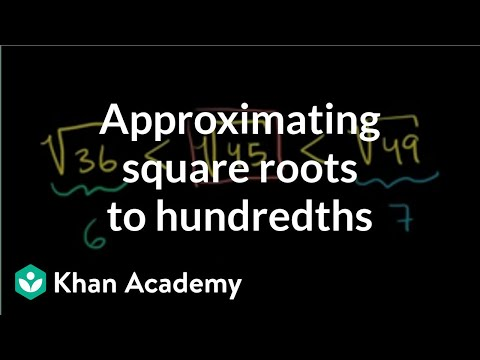Video

# Approximating square roots (Full video)

Description: Learn how to find the approximate values of the square roots of 32, 55, and 123. The technique used is to compare the squares of whole numbers to the number we're taking the square root of. What I want to do in this video is get a little bit of experience, see a few examples of trying to roughly estimate the square root of non-perfect squares. So let's say that I had, if I wanted to estimate the square root of 32. And in particular, I'm just curious, between what two integers will this square root lie?

### Other videos you might be interested in### Approximating square roots to hundredths (Full video)